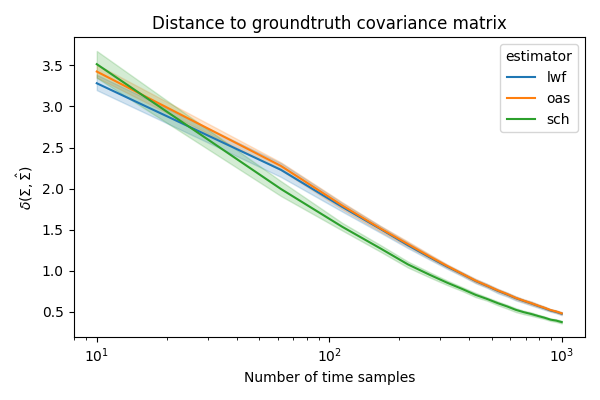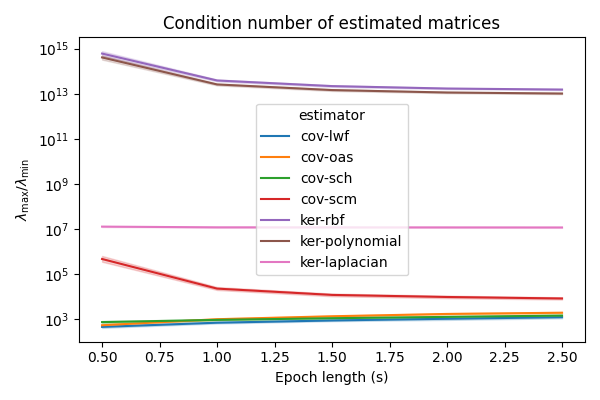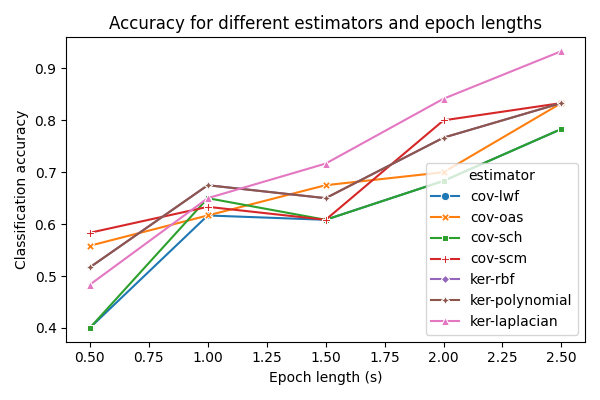# Compare covariance and kernel estimators with different time windows¶

Comparison of covariance estimators for different EEG signal lengths and their impact on classification . Kernel estimators are also compared .

# Authors: Sylvain Chevallier and Quentin Barthélemy
#

import numpy as np
import pandas as pd
from matplotlib import pyplot as plt
import seaborn as sns

from mne import Epochs, pick_types, events_from_annotations
from mne.io import concatenate_raws
from mne.datasets import eegbci
from sklearn.model_selection import cross_val_score, StratifiedKFold
from sklearn.pipeline import make_pipeline

from pyriemann.estimation import Covariances, Kernels
from pyriemann.utils.distance import distance
from pyriemann.classification import MDM


## Estimating covariance on synthetic data¶

Generate synthetic data, sampled from a distribution considered as the groundtruth.

rs = np.random.RandomState(42)
n_matrices, n_channels, n_times = 10, 5, 1000
var = 2.0 + 0.1 * rs.randn(n_matrices, n_channels)
A = 2 * rs.rand(n_channels, n_channels) - 1
A /= np.linalg.norm(A, axis=1)[:, np.newaxis]
true_covs = np.empty(shape=(n_matrices, n_channels, n_channels))
X = np.empty(shape=(n_matrices, n_channels, n_times))
for i in range(n_matrices):
true_covs[i] = A @ np.diag(var[i]) @ A.T
X[i] = rs.multivariate_normal(
np.array([0.0] * n_channels), true_covs[i], size=n_times
).T


Covariances() class offers several estimators:

• sample covariance matrix (SCM),

• Ledoit-Wolf (LWF),

• Schaefer-Strimmer (SCH),

• oracle approximating shrunk (OAS) covariance,

• minimum covariance determinant (MCD),

• and others.

We will compare the distance of LWF, OAS and SCH estimators with the groundtruth, while increasing epoch length.

estimators = ["lwf", "oas", "sch"]
w_len = np.linspace(10, n_times, 20, dtype=int)
dfd = list()
for est in estimators:
for wl in w_len:
est_covs = Covariances(estimator=est).transform(X[:, :, :wl])
dists = distance(est_covs, true_covs, metric="riemann")
dfd.extend([dict(estimator=est, wlen=wl, dist=d) for d in dists])
dfd = pd.DataFrame(dfd)

fig, ax = plt.subplots(figsize=(6, 4))
ax.set(xscale="log")
sns.lineplot(data=dfd, x="wlen", y="dist", hue="estimator", ax=ax)
ax.set_title("Distance to groundtruth covariance matrix")
ax.set_xlabel("Number of time samples")
ax.set_ylabel(r"$\delta(\Sigma, \hat{\Sigma})$")
plt.tight_layout()
plt.show()## Choice of estimator for motor imagery data¶

event_id = dict(hands=2, feet=3)
subject = 1
runs = [6, 10, 14]  # motor imagery: hands vs feet
raw_files = [
]
raw = concatenate_raws(raw_files)

# subsample elecs
picks = picks[::2]
# Apply band-pass filter
raw.filter(7.0, 35.0, method="iir", picks=picks, skip_by_annotation="edge")
events, _ = events_from_annotations(raw, event_id=dict(T1=2, T2=3))
event_ids = dict(hands=2, feet=3)

Extracting EDF parameters from /home/docs/mne_data/MNE-eegbci-data/files/eegmmidb/1.0.0/S001/S001R06.edf...
EDF file detected
Setting channel info structure...
Creating raw.info structure...
Reading 0 ... 19999  =      0.000 ...   124.994 secs...
Extracting EDF parameters from /home/docs/mne_data/MNE-eegbci-data/files/eegmmidb/1.0.0/S001/S001R10.edf...
EDF file detected
Setting channel info structure...
Creating raw.info structure...
Reading 0 ... 19999  =      0.000 ...   124.994 secs...
Extracting EDF parameters from /home/docs/mne_data/MNE-eegbci-data/files/eegmmidb/1.0.0/S001/S001R14.edf...
EDF file detected
Setting channel info structure...
Creating raw.info structure...
Reading 0 ... 19999  =      0.000 ...   124.994 secs...
Filtering a subset of channels. The highpass and lowpass values in the measurement info will not be updated.
Filtering raw data in 3 contiguous segments
Setting up band-pass filter from 7 - 35 Hz

IIR filter parameters
---------------------
Butterworth bandpass zero-phase (two-pass forward and reverse) non-causal filter:
- Filter order 16 (effective, after forward-backward)
- Cutoffs at 7.00, 35.00 Hz: -6.02, -6.02 dB

Used Annotations descriptions: ['T1', 'T2']


## Influence of shrinkage to estimate matrices¶

Sample covariance matrix (SCM) estimation could lead to ill-conditionned matrices depending on the quality and quantity of EEG data available. Matrix condition number is the ratio between the highest and lowest eigenvalues: high values indicates ill-conditionned matrices that are not suitable for classification. A common approach to mitigate this issue is to regularize covariance matrices by shrinkage, like in Ledoit-Wolf, Schaefer-Strimmer or oracle estimators.

In addition to covariance matrices, kernel matrices are computed for three kernel functions:

• polynomial,

• Laplacian.

estimators = [
"cov-lwf", "cov-oas", "cov-sch", "cov-scm",
"ker-rbf", "ker-polynomial", "ker-laplacian",
]
tmin = -0.2
w_len = np.linspace(0.2, 2, 10)
n_matrices = 45
dfc = list()

for wl in w_len:
X = Epochs(
raw,
events,
event_ids,
tmin,
tmin + wl,
picks=picks,
verbose=False,
).get_data()
for est in estimators:
est_class, est_param = est.split('-')
if est_class == "ker":
covs = Kernels(metric=est_param).transform(X)
else:
covs = Covariances(estimator=est_param).transform(X)
evals, _ = np.linalg.eigh(covs)
dfc.extend([dict(estimator=est, wlen=wl, cond=e[-1] / e)
for e in evals])
dfc = pd.DataFrame(dfc)

fig, ax = plt.subplots(figsize=(6, 4))
ax.set(yscale="log")
sns.lineplot(data=dfc, x="wlen", y="cond", hue="estimator", ax=ax)
ax.set_title("Condition number of estimated matrices")
ax.set_xlabel("Epoch length (s)")
ax.set_ylabel(r"$\lambda_{\max}$/$\lambda_{\min}$")
plt.tight_layout()
plt.show()## Picking a good estimator for classification¶

The choice of estimator have an impact on classification, especially when the matrices are estimated on short time windows.

tmin = 0.0
w_len = np.linspace(0.2, 2.0, 5)
n_matrices, n_splits = 45, 5
dfa = list()
sc = "balanced_accuracy"

cv = StratifiedKFold(n_splits=n_splits, shuffle=True, random_state=123)
for wl in w_len:
epochs = Epochs(
raw,
events,
event_ids,
tmin,
tmin + wl,
proj=True,
picks=picks,
baseline=None,
verbose=False,
)
X = epochs.get_data()
y = np.array([0 if ev == 2 else 1 for ev in epochs.events[:, -1]])
for est in estimators:
est_class, est_param = est.split('-')
if est_class == "ker":
clf = make_pipeline(Kernels(metric=est_param), MDM())
else:
clf = make_pipeline(Covariances(estimator=est_param), MDM())
try:
score = cross_val_score(clf, X, y, cv=cv, scoring=sc)
dfa += [dict(estimator=est, wlen=wl, accuracy=sc) for sc in score]
except ValueError:
print(f"{est}: {wl} is not sufficent to estimate a SPD matrix")
dfa += [dict(estimator=est, wlen=wl, accuracy=np.nan)] * n_splits
dfa = pd.DataFrame(dfa)

/home/docs/checkouts/readthedocs.org/user_builds/pyriemann/checkouts/latest/pyriemann/utils/base.py:18: RuntimeWarning: invalid value encountered in log
eigvals = operator(eigvals)
cov-scm: 0.2 is not sufficent to estimate a SPD matrix
/home/docs/checkouts/readthedocs.org/user_builds/pyriemann/checkouts/latest/pyriemann/utils/base.py:18: RuntimeWarning: invalid value encountered in log
eigvals = operator(eigvals)
/home/docs/checkouts/readthedocs.org/user_builds/pyriemann/checkouts/latest/pyriemann/utils/base.py:18: RuntimeWarning: invalid value encountered in log
eigvals = operator(eigvals)
/home/docs/checkouts/readthedocs.org/user_builds/pyriemann/checkouts/latest/pyriemann/utils/base.py:18: RuntimeWarning: invalid value encountered in log
eigvals = operator(eigvals)
/home/docs/checkouts/readthedocs.org/user_builds/pyriemann/checkouts/latest/pyriemann/utils/base.py:18: RuntimeWarning: invalid value encountered in log
eigvals = operator(eigvals)
/home/docs/checkouts/readthedocs.org/user_builds/pyriemann/checkouts/latest/pyriemann/utils/base.py:18: RuntimeWarning: invalid value encountered in log
eigvals = operator(eigvals)
ker-rbf: 0.2 is not sufficent to estimate a SPD matrix
/home/docs/checkouts/readthedocs.org/user_builds/pyriemann/checkouts/latest/pyriemann/utils/base.py:18: RuntimeWarning: invalid value encountered in log
eigvals = operator(eigvals)
/home/docs/checkouts/readthedocs.org/user_builds/pyriemann/checkouts/latest/pyriemann/utils/base.py:18: RuntimeWarning: invalid value encountered in log
eigvals = operator(eigvals)
/home/docs/checkouts/readthedocs.org/user_builds/pyriemann/checkouts/latest/pyriemann/utils/base.py:18: RuntimeWarning: invalid value encountered in log
eigvals = operator(eigvals)
/home/docs/checkouts/readthedocs.org/user_builds/pyriemann/checkouts/latest/pyriemann/utils/base.py:18: RuntimeWarning: invalid value encountered in log
eigvals = operator(eigvals)
/home/docs/checkouts/readthedocs.org/user_builds/pyriemann/checkouts/latest/pyriemann/utils/base.py:18: RuntimeWarning: invalid value encountered in log
eigvals = operator(eigvals)
ker-polynomial: 0.2 is not sufficent to estimate a SPD matrix
warnings.warn("Convergence not reached")
warnings.warn("Convergence not reached")
warnings.warn("Convergence not reached")
warnings.warn("Convergence not reached")
warnings.warn("Convergence not reached")
warnings.warn("Convergence not reached")
warnings.warn("Convergence not reached")
warnings.warn("Convergence not reached")
warnings.warn("Convergence not reached")
warnings.warn("Convergence not reached")
warnings.warn("Convergence not reached")
warnings.warn("Convergence not reached")
warnings.warn("Convergence not reached")
warnings.warn("Convergence not reached")
warnings.warn("Convergence not reached")
warnings.warn("Convergence not reached")
warnings.warn("Convergence not reached")
warnings.warn("Convergence not reached")
warnings.warn("Convergence not reached")
warnings.warn("Convergence not reached")
warnings.warn("Convergence not reached")
warnings.warn("Convergence not reached")
warnings.warn("Convergence not reached")
warnings.warn("Convergence not reached")
warnings.warn("Convergence not reached")
warnings.warn("Convergence not reached")
warnings.warn("Convergence not reached")
warnings.warn("Convergence not reached")
warnings.warn("Convergence not reached")
warnings.warn("Convergence not reached")
warnings.warn("Convergence not reached")
warnings.warn("Convergence not reached")
warnings.warn("Convergence not reached")
warnings.warn("Convergence not reached")
warnings.warn("Convergence not reached")
warnings.warn("Convergence not reached")
warnings.warn("Convergence not reached")
warnings.warn("Convergence not reached")
warnings.warn("Convergence not reached")
warnings.warn("Convergence not reached")

fig, ax = plt.subplots(figsize=(6, 4))
sns.lineplot(
data=dfa,
x="wlen",
y="accuracy",
hue="estimator",
style="estimator",
ax=ax,
ci=None,
markers=True,
dashes=False,
)
ax.set_title("Accuracy for different estimators and epoch lengths")
ax.set_xlabel("Epoch length (s)")
ax.set_ylabel("Classification accuracy")
plt.tight_layout()
plt.show()/home/docs/checkouts/readthedocs.org/user_builds/pyriemann/checkouts/latest/examples/signal/plot_covariance_estimation.py:214: FutureWarning:

The ci parameter is deprecated. Use errorbar=None for the same effect.

sns.lineplot(